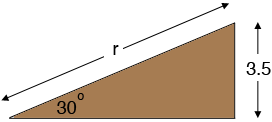SEARCH HOMEMath Central Quandaries & QueriesQuestion from Jaylynn, a student: Hi, I've been trying to figure out how long my ramp would have to be in order to reach a height of 3.5 feet at a 30 degree angle for a sugar glider enclosure?Hi Jaylynn,

Suppose the length of the ramp is $r$ feet then I get the diagramThe ramp forms a right triangle and the sine of the $30^o$ angle is thus $\frac{3.5}{r}.$ But the sine of a $30^o$ angle is $\frac12$ and thus

$\frac{3.5}{r} = \frac12 .$

Threrfore $r = 2 \times 3.5 = 7$ feet.

PennyMath Central is supported by the University of Regina and the Imperial Oil Foundation.